Chemical Bonding - Main Group - Periodic Properties

# Chemical Bonding - Main Group - Periodic Properties

Test Description

## 60 Questions MCQ Test Mock Test Series for IIT JAM Chemistry | Chemical Bonding - Main Group - Periodic Properties

Chemical Bonding - Main Group - Periodic Properties for Chemistry 2022 is part of Mock Test Series for IIT JAM Chemistry preparation. The Chemical Bonding - Main Group - Periodic Properties questions and answers have been prepared according to the Chemistry exam syllabus.The Chemical Bonding - Main Group - Periodic Properties MCQs are made for Chemistry 2022 Exam. Find important definitions, questions, notes, meanings, examples, exercises, MCQs and online tests for Chemical Bonding - Main Group - Periodic Properties below.
Solutions of Chemical Bonding - Main Group - Periodic Properties questions in English are available as part of our Mock Test Series for IIT JAM Chemistry for Chemistry & Chemical Bonding - Main Group - Periodic Properties solutions in Hindi for Mock Test Series for IIT JAM Chemistry course. Download more important topics, notes, lectures and mock test series for Chemistry Exam by signing up for free. Attempt Chemical Bonding - Main Group - Periodic Properties | 60 questions in 180 minutes | Mock test for Chemistry preparation | Free important questions MCQ to study Mock Test Series for IIT JAM Chemistry for Chemistry Exam | Download free PDF with solutions
 1 Crore+ students have signed up on EduRev. Have you?
Chemical Bonding - Main Group - Periodic Properties - Question 1

### The inter-nuclear distance in O—O bonds for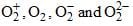respectively are:

Chemical Bonding - Main Group - Periodic Properties - Question 2

### Arrange the following compounds in their C—H bond length:            (I) CH3F      (II) CH2F2       (III) CHF3

Chemical Bonding - Main Group - Periodic Properties - Question 3

### Arrange the following species in increasing order of stability:            Li2, Li2+, Li2–

Chemical Bonding - Main Group - Periodic Properties - Question 4

Which of the following is correct structure of S2Cl2?

Chemical Bonding - Main Group - Periodic Properties - Question 5

Elements X and Y have valence shell electronic configuration as:
X: ns2np    Y: ns2np3
Which compound is likely to be formed?

Chemical Bonding - Main Group - Periodic Properties - Question 6

M+3 has electronic configuration as [Ar]3d104s2, hence it lies in following block:

Detailed Solution for Chemical Bonding - Main Group - Periodic Properties - Question 6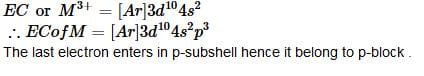Chemical Bonding - Main Group - Periodic Properties - Question 7

Pick out the property which is not shown by transition metals:

Chemical Bonding - Main Group - Periodic Properties - Question 8

Which one is correct: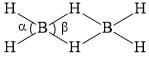Chemical Bonding - Main Group - Periodic Properties - Question 9

Which among the following is both a molecule and a compound?

Detailed Solution for Chemical Bonding - Main Group - Periodic Properties - Question 9

H2O is both a molecule and a compound. This is because the atoms which make them are not the same.

Chemical Bonding - Main Group - Periodic Properties - Question 10

Standard reduction potentials in acidic medium for F2, Cl2, Na, Zn are in the order:

Chemical Bonding - Main Group - Periodic Properties - Question 11

Consider the compounds PF5, SbF5, PH3 and SbH3. The strongest acid and strongest base among these are resp:

Chemical Bonding - Main Group - Periodic Properties - Question 12

Among SiCl4, P(O)Cl3, NF3, trans-[SnCl4(py)2] (py = pyridine), those with zero dipole moments are:

Chemical Bonding - Main Group - Periodic Properties - Question 13

The nodal plane in the π-bond of ethene is located in:

Chemical Bonding - Main Group - Periodic Properties - Question 14

Ionic hydrides reacts with water to give

Chemical Bonding - Main Group - Periodic Properties - Question 15

Consider (I) I-, (II) Se2-, (III) Br, (IV) O2-, (V) F- and arrange them in decreasing order of ionic radius.

Chemical Bonding - Main Group - Periodic Properties - Question 16

Which pair is different from others?

Chemical Bonding - Main Group - Periodic Properties - Question 17

The d-bond is formed via the overlap of (considering z-axis as the inter-nuclear axis):

Chemical Bonding - Main Group - Periodic Properties - Question 18

First, Second and Third ionization energy values are 100 eV, 150 eV and 1500 eV. Element can be?

Chemical Bonding - Main Group - Periodic Properties - Question 19

Which of the following compunds is not formed by all alkali metals?

Detailed Solution for Chemical Bonding - Main Group - Periodic Properties - Question 19

All alkali metals form hydrides, hydroxides and amide but metals like Li and Na do not from superoxide MO2.

Chemical Bonding - Main Group - Periodic Properties - Question 20

Least acidic tri-halide is?

Chemical Bonding - Main Group - Periodic Properties - Question 21

What are the hydrolysis products of calcium carbide?

Chemical Bonding - Main Group - Periodic Properties - Question 22

Noble gases (like He, Ne, Ar, Kr etc) are isolated from air. One of the steps is/are

Chemical Bonding - Main Group - Periodic Properties - Question 23

To an acidified dichromate solution, a pinch of Na2O2 and ether is added and shaken. What is the observation?

Chemical Bonding - Main Group - Periodic Properties - Question 24

The reason for the chemical inertness of gaseous nitrogen at room temperature is best given by its:

Chemical Bonding - Main Group - Periodic Properties - Question 25

The correct increasing order of melting points of the following is:

Chemical Bonding - Main Group - Periodic Properties - Question 26

Chemical Bonding - Main Group - Periodic Properties - Question 27

Which represents Alkali metals based on IE1and IE2 values?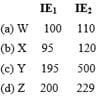Chemical Bonding - Main Group - Periodic Properties - Question 28

Chemical Bonding - Main Group - Periodic Properties - Question 29

Which has maximum stability?

Chemical Bonding - Main Group - Periodic Properties - Question 30

Which is best oxidizing agent?

Chemical Bonding - Main Group - Periodic Properties - Question 31

An atom differs from its ion in which among the following ?

Detailed Solution for Chemical Bonding - Main Group - Periodic Properties - Question 31

When an atom loses or gains electrons its forms its corresponding ion, and hence it differs in number of electrons.

For example, H atom has 1 electron. Whereas H+ ion has 0 electrons. On the other hand, H ion has 2 electrons.

*Multiple options can be correct
Chemical Bonding - Main Group - Periodic Properties - Question 32

Select correct statement(s)

*Multiple options can be correct
Chemical Bonding - Main Group - Periodic Properties - Question 33

Which of the following statement(s) is/are correct?

*Multiple options can be correct
Chemical Bonding - Main Group - Periodic Properties - Question 34

Which of the following will give H2O2 on hydrolysis?

*Multiple options can be correct
Chemical Bonding - Main Group - Periodic Properties - Question 35

Which statement is/are correct?

*Multiple options can be correct
Chemical Bonding - Main Group - Periodic Properties - Question 36

Select correct statement(s):

*Multiple options can be correct
Chemical Bonding - Main Group - Periodic Properties - Question 37

Which of the following statement(s) is/are true?

*Multiple options can be correct
Chemical Bonding - Main Group - Periodic Properties - Question 38

For the process, select the correct alternate:
X(g) + e → X(g),     ∆H = x
X–(g) →X(g) + e,     ∆H = y

*Multiple options can be correct
Chemical Bonding - Main Group - Periodic Properties - Question 39

Which pair represent/s correct first IE?​

*Multiple options can be correct
Chemical Bonding - Main Group - Periodic Properties - Question 40

Select correct alternate(s):

*Answer can only contain numeric values
Chemical Bonding - Main Group - Periodic Properties - Question 41

The number of pairs which shows correct order of angle:

(I) NH3 > PH3                         (II) H2S < H2O               (III) NO2+ > NO2               (IV) NO2+ > NO2
(V) NO2> NO2+                    (VI) Cl2O < OF2            (VII) H2O > OF2

*Answer can only contain numeric values
Chemical Bonding - Main Group - Periodic Properties - Question 42

Total number of compound(s) having ‘X-X’ linkage. (where X is 15th group element):
N2O4, N2O, P4O6, N2O3, N2H4, P4S10, N2O5, H4P2O6, H2N2O2

*Answer can only contain numeric values
Chemical Bonding - Main Group - Periodic Properties - Question 43

Find number of interstitial carbides among following:
V2C, V4C3, CaC2, Mg2C3, SiC, B4C, Al4C3, WC, V6C5, TiC, Cr3C2, Nb4C3, Mo3C2.

*Answer can only contain numeric values
Chemical Bonding - Main Group - Periodic Properties - Question 44

The bond angle in PBr3 is 101°. The percentage ‘s’ character of the central atom is: [upto 2 decimal place]

*Answer can only contain numeric values
Chemical Bonding - Main Group - Periodic Properties - Question 45

Calculate the total number of possible resonating structures of HN3 and NaN3 [provide combined value]:

*Answer can only contain numeric values
Chemical Bonding - Main Group - Periodic Properties - Question 46

For Na2B4O7.10H2O, determine the value of X + Y + Z
X = Total number of water molecules of crystallization
Y = Total number of B—O—B bonds
Z = Total number of tetrahedral boron atoms

*Answer can only contain numeric values
Chemical Bonding - Main Group - Periodic Properties - Question 47

Find the number of superoxides among following: Li2O, Na2O2, KO2, RbO2, CsO2

*Answer can only contain numeric values
Chemical Bonding - Main Group - Periodic Properties - Question 48

Maximum oxidation state shown by Mn is?

*Answer can only contain numeric values
Chemical Bonding - Main Group - Periodic Properties - Question 49

Electronegativity = [IE + EA]/N. This ‘N’ is?

*Answer can only contain numeric values
Chemical Bonding - Main Group - Periodic Properties - Question 50

Catenation is maximum for the element with atomic number?

*Answer can only contain numeric values
Chemical Bonding - Main Group - Periodic Properties - Question 51

Among the following the number of compounds/ions having a linear structure is: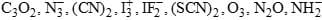*Answer can only contain numeric values
Chemical Bonding - Main Group - Periodic Properties - Question 52

The total number of lone pairs of electrons in N2O3 is:

*Answer can only contain numeric values
Chemical Bonding - Main Group - Periodic Properties - Question 53

The dipole moment of HCl is 1.03 D, if H—Cl bond distance is 1.26 Å, what is the percentage of ionic character in the H—Cl bond?

*Answer can only contain numeric values
Chemical Bonding - Main Group - Periodic Properties - Question 54

The molecule MLx is planar with 7 pairs of electrons around M in the valence shell. The value of x is:

*Answer can only contain numeric values
Chemical Bonding - Main Group - Periodic Properties - Question 55

In complete hydrolysis of P4S6, how many water molecules are required?

*Answer can only contain numeric values
Chemical Bonding - Main Group - Periodic Properties - Question 56

Consider the following reaction: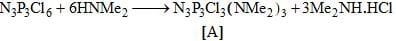The number of possible isomers for [A] is:

*Answer can only contain numeric values
Chemical Bonding - Main Group - Periodic Properties - Question 57

How many oxygen atoms are shared in Pyroxenes?

*Answer can only contain numeric values
Chemical Bonding - Main Group - Periodic Properties - Question 58

Number of unpaired electrons n Gd(64) is?

*Answer can only contain numeric values
Chemical Bonding - Main Group - Periodic Properties - Question 59

Maximum oxidation state shown by Chalcogens is?

*Answer can only contain numeric values
Chemical Bonding - Main Group - Periodic Properties - Question 60

How many of following silicates are Neso-silicates?
Be2SiO4, Zn2SiO4, Sc2SiO7, ZrSiO4, Mg2SiO4, LiAl(SiO3)2, Fe2SiO4, Mg3Si4O10(OH)2

## Mock Test Series for IIT JAM Chemistry

2 docs|25 tests
 Use Code STAYHOME200 and get INR 200 additional OFF Use Coupon Code
Information about Chemical Bonding - Main Group - Periodic Properties Page
In this test you can find the Exam questions for Chemical Bonding - Main Group - Periodic Properties solved & explained in the simplest way possible. Besides giving Questions and answers for Chemical Bonding - Main Group - Periodic Properties, EduRev gives you an ample number of Online tests for practice

2 docs|25 tests